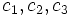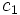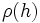# Size-degree-weighted characters are algebraic integers

## Statement

### For an algebraically closed field of characteristic zero

Suppose$k$ is an algebraically closed field of characteristic zero, and$G$ is a finite group. Let$\rho$ be an Irreducible linear representation (?) of$G$ over$k$, and$\chi$ be the character corresponding to$\rho$. Let$c$ be a conjugacy class in$G$ and$g \in c$ be an element. Then:$\frac{|c|\chi(g)}{\chi(1)}$

is an algebraic integer.

Note that both aspects: irreducibility of the representation and the fact that we are working over an algebraically closed field of characteristic zero, are required. Getting rid of either condition makes the statement false.

### For a splitting field

We can weaken the condition on$k$ somewhat: instead of requiring it to be an algebraically closed field of characteristic zero, it suffices to require$k$ to be a Splitting field (?) for$G$. In particular, any sufficiently large field for$G$ will do.

## Related facts

### Breakdown for a field that is not algebraically closed

Further information: cyclic group:Z3

Let$G$ be the cyclic group of order three and$\R$ be the field.$G$ has an irreducible two-dimensional linear representation over$\R$ given by rotation by multiples of$2\pi/3$. For a non-identity element$g$ of$G$,$\chi(g) = -1$ for the corresponding character, while$\chi(1) = 2$. Thus, the expression works out to$-1/2$, which is not an algebraic integer.

### Breakdown for a representation that is not irreducible

The same example as the above (the one for breakdown over a field that is not algebraically closed) works. Specifically, the irreducible representation over$\R$ can be viewed as a reducible representation over$\mathbb{C}$.

## Proof

The proof is based on the idea of the convolution algebra on conjugacy classes.

### Description of the convolution algebra on conjugacy classes

Let$C(G,\mathbb{Z})$ be a$\mathbb{Z}$-subalgebra of the group ring$\mathbb{Z}(G)$ defined as follows: as a group, it is the free Abelian group on all indicator class functions for conjugacy classes. In other words, for each conjugacy class, we have a free generator that corresponds to the sum of elements of that conjugacy class.

The structure constant for multiplication of elements of$C(G,\mathbb{Z})$ is defined as follows: given conjugacy classes$c_1, c_2, c_3$, the coefficient of the$c_3$-indicator function in the product of the$c_1$-indicator function and the$c_2$-indicator function is the number of ways of writing$g_1g_2 = a$ where$g_i \in c_i$, and$a$ a fixed element of$c_3$.

Note that all the structure constants are integers.

### A homomorphism from this convolution algebra to the matrix ring

The representation$\rho$ gives rise to a homomorphism from$C(G,\mathbb{Z})$ to the matrix ring$M_n(k)$. The indicator function for a conjugacy class$c$ goes to the matrix given by:$\sum_{g \in c} \rho(g)$.

This sum commutes with$\rho(h)$ for all$h$, and thus, by Schur's lemma, the sum is a scalar matrix. The trace of the sum is$|c|\chi(g)$, so the sum must be a scalar matrix with scalar entry:$\frac{|c|\chi(g)}{\chi(1)}$.

Thus, the set of scalar matrices with entries described as above additively generate a group that is a ring under multiplication. The structure constants for this ring are the same as the structure constants for the convolution algebra. A result from algebraic number theory now tells us that this forces the entire ring to be a ring of algebraic integers, and in particular, the generating elements are algebraic integers.